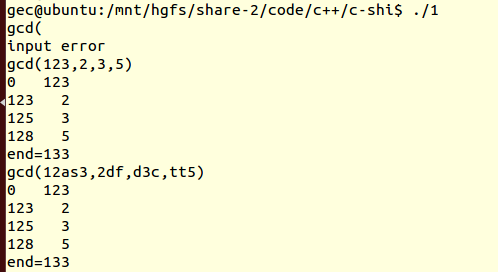2019-11-13 16:37

# C语言如何提取命令行输入的字符串中的整数，并传递到自定义函数？

15
• c语言

⭐**在函数中提取m,n的值并计算结果？ （因为有多个整形数值，并且有个','所以这种下面写法无法实现取值。。。。**

``````    char str, digit;
char* ps;
int i = 0;

ps = str;
while (*ps != '\0')
{
if (*ps >= '0' && *ps <= '9' || ps==',')
{
digit[i] = *ps;    //找到数字，存放到数组digit里
i++;
}
ps++;    //ps指针指向字符串下一个字符
}
digit[i] = '\0';    //为了能用%s输出，digit在末尾要加一个结束字符'\0'
printf("%s\n", digit);
```）

``

``````

**如果要传递m,n,o,q多个整形数据呢？

**

`````` int main(argc,argv[])

char* stdinin = NULL;
stdinin = ufgets(stdin);       //ufgets是自定义函数，类似于fgets
//然后是一些判断是否输入为空等
if (strncmp(stdinin, "gcd", 3) == 0)
gcd(m, n);
//----函数-----
void gcd(n, m）
{
这里并不能直接使用stdinin

}
``````
• 点赞
• 写回答
• 关注问题
• 收藏
• 复制链接分享
• 邀请回答

#### 1条回答

• 代码我写了，如果有其他需求需要修改一个也没什么，可以很快修改，我也写了详细的注释
还有疑惑也可以交流，如果不是需求，应该也是小的改动。

下面是几次的测试，环境是gcc的，为了方便检查，输出我加入了运算过程
，不需要，你可以去掉，希望能对你有所帮助，空闲时间有点少，写的也就有点乱。``````#include <stdio.h>
#include <stdlib.h>
#include<string.h>
int  gcd(int i,int j)//你需要执行的函数，可以换的
{
printf("%d   %d\n",i,j);
return i+j;
}
int change(char *str)//将一串字符串转换成一个数字
{
int a={0};
int i=0;
int j=0;
int num=0;
while(str[i])
{
if((str[i]>=48)&&str[i]<=57)
{
a[j]=str[i]-48;
j++;
}
i++;
}
for(i=0;i<j;i++)
{
num=num*10+a[i];
}
return num;
}
int main()
{
char s1,s2;
char now_time;
int i,j,key,n,m;
while(1)
{
memset(s1,0,256);
memset(s2,0,256);
memset(now_time,0,256);//每次对三个数组进行清零，如果没有学过可以查一下，也是string的基本函数
key=n=m=0;
scanf("%s",s1);//输入字符串
j=strlen(s1);
if((s1!='g')|(s1!='c')|(s1!='d')|(s1!='(')|(s1[j-1]!=')'))//针对输出格式错误的采取报错忽略
{
printf("input error\n");
continue;
}
s1[j+1]=s1[j];
s1[j]=',';//在最后一个自动添加一个‘，’号，确保每个‘，’前均是一个操作数
strcpy(s1,&s1);//将输入字符串前进4格，去除前面的格式
j=strlen(s1);//记录字符串长度，作为后面的结束的判断标志
for(i=0;i<=j;i++)
{
if(s1[i]!=',')//获取第一个操作数
{
now_time[n++]=s1[i];
}
else
{
n=change(now_time);//将操作数由字符串转为数字
m=gcd(m,n);//进行操作，第一次m为默认0，你可以自己设置其他不影响gcd函数的值
//当然你也可以通过加一些其他修改，这样写只是为了方面代码实现，后面的m均表示前面的数字的运算和
n=0;//操作数清零，等待下一次操作
memset(now_time,0,256);//获取操作数字符串清零
}

}
printf("end=%d\n",m);//输出最后运算结果
}
return 0;
}

``````

ss

点赞 3 评论 复制链接分享